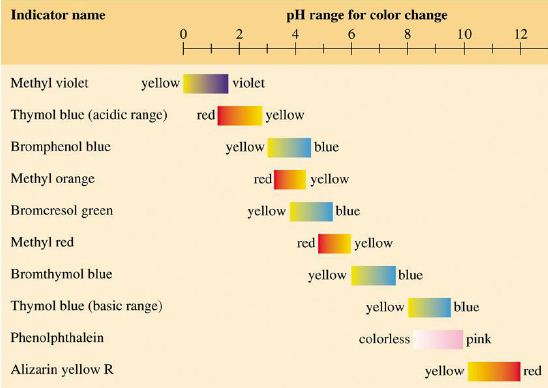# Measuring pH Solution

The pH of a solution can accurately be measured using a pH meter.

Although less precise, acid-base indicators are often used to measure pH because they usually change colour within a narrow pH range. Litmus is a common indicator which is red in acid and blue in alkali. There are many other acid-base indicators which change colour at various pH values as shown in the Figure below.KW is temperature dependent and has a value of 1.0 x 10-14 at 25°C. The pH scale is based on the [H+] which is related to the pH by

pH = – log [H+]

This relationship can be used to calculate the concentration of H+ ions and OH ions since changes in the concentration of one directly change the concentration of the other to maintain a constant KW value.

The pOH of a solution is defined as the negative logarithm of the molar hydroxide- ion concentration as follows:

pOH = -log10 [OH-]